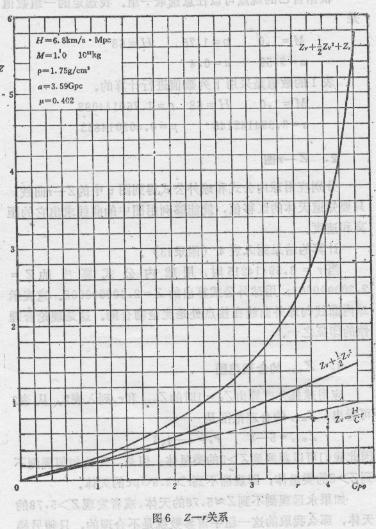(2)  Z-r  diagram

We apply the formulae outside the sphere as well as the formulae inside the sphere to obtain Z-r diagram (Fig. 4.). As long as the redshift of galaxy is known, its distance and velocity can be found in the Fig. 6.

Fig.6  Z-r diagram

Zr